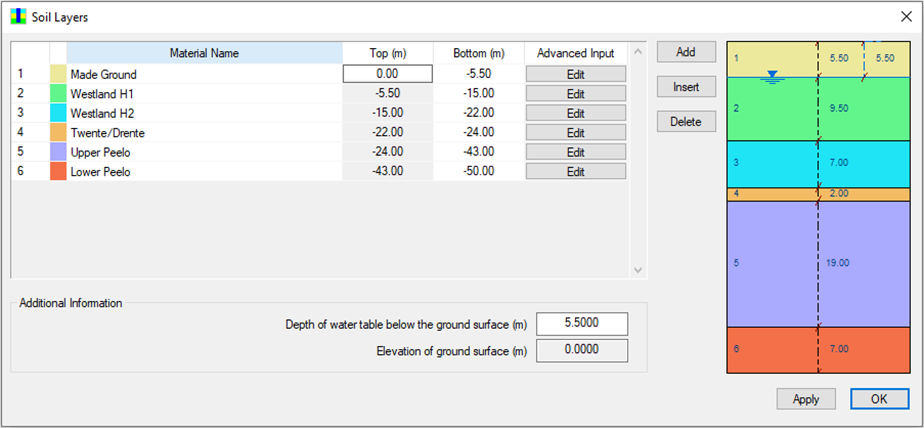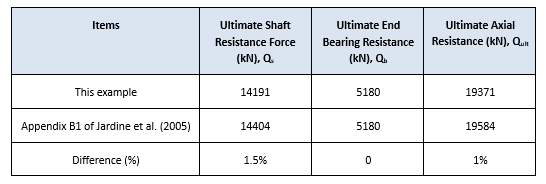top of page

# EURIPIDES steel pipe test pile driven into fine to medium sands

This blog presents a case study for an example documented in Appendix B1 of the book of "ICP Design Methods for Driven Piles in Sands and Clays” by Jardine et al. (2005) using the PileAXL program. This case is part of the testing programme selected by the Joint-Industry EURIPIDES project for large diameter pile tests in dense sand at Eemshaven in the Netherlands.Figure 1 Ground profile at EURIPIDES testing site (after Jardine et al., 2005)

The ground profile of the site consists of about 5 m thick made ground (fine sand) followed by Holocene and Pleistocene fine to medium sands with the thickness of greater than 60 m. Figure 1 shows the general soil profile through the site and the cone penetration testing records. This example is based on the profile of CPT36 which is shown in more detail on Figure 2.Figure 2 CPT 36 profile at EURIPIDES testing site (after Jardine et al., 2005)

The test pile was a nominal 30’’OD x 1.5’’WT steel pipe pile which was driven 47 m into the ground. The outside diameter is 763 mm and the inside diameter is 691 mm. The pile surface roughness is 0.025 mm. Jardine et al (2005) provides the cone tip resistance values at 1 m interval in the axial capacity calculation for this test pile. Those cone tip resistance values are extracted and tabulated in the excel spreadsheet as shown in Figure 3 and saved into a CSV file. The depth values (in unit of meter) are under the column “A”. The cone tip resistance values are under the column “B”.Figure 3 CPT 36 profile summarised in the excel spreadsheet file

This CSV file is then imported into the PileAXL program through the function of CPTu File Manager built into the program. Figure 4 shows the CPT profile imported into the PileAXL program. Note that there are no plots for fs, u2 and Rf because only cone tip resistance values are imported and used in the calculation. Other parameters are not required.Figure 4 CPT profile imported into the PileAXL program

Apart from the cone tip resistance values, the application of ICP sand method also needs other parameters such as unit weight and constant volume interface angle of friction. For the axial capacity calculation with the PileAXL program, the ground profile is therefore simplified into 6 different soil units and the input parameters are summarized in the table below based on the calculation information presented in Jardine et al. (2005).Table 1 Soil layer input parameters

The ground profile input dialog for this case is shown in Figure 5. The groundwater table is adopted to be at the bottom of the first soil layer – Made Ground, which is 5.5 m below the ground surface.Figure 5 Soil layer and groundwater table input parameters

Figure 6 shows the ground profile with the pile length and loading conditions for this example. Note that the CPT profile is also automatically displayed together with the soil layers because the CPT profile is adopted in the calculation for this example.Figure 7 shows the input parameters for the pipe pile section. The outside diameter of the section diameter is 0.763 m. The inside diameter is 0.691 mm. The Young’s modulus of the steel pile is adopted to be 200 GPa. Note that this parameter is required for the pile settlement analysis where the elastic pile shortening under the axial load at the pile head can be calculated. The advanced option for pipe section is set as “open-ended pipe pile” as the steel tubular pile in this example is driven with an open end.Figure 7 Section input parameters for 763 mm OD X 36 mm thickness pipe pile

Figure 8 shows the typical input parameters for one of the soil layers - Westland H1 soil material which is modelled with “ICP Sand” model. The unit weight for this layer is 19.5 kN/m^3. The constant volume interface angle of friction is 29 degree.Figure 8 Input parameters for Westland H1 material

Analysis option input parameters for this example are shown in Figure 9. The pile type is selected to be “Driven Pile”. The maximum pile length is set as 47 m and the length increment number is adopted as 94 to have the pile element of 0.5 m. The axial loading for settlement analysis is adopted to be 18750 kN to calculate the load settlement curve. Working stress design approach is adopted to be the pile capacity design code. The pile surface roughness parameter is adopted to be 1.25 x 10^-5 m which gives the pile surface roughness of 0.025 mm.Figure 9 Analysis option input parameters

Figure 10 shows the general output window of the PileAXL program for this example. Three axial capacity plot items and the tabulated results for the selected pile section are presented in the output window.Figure 10 General axial capacity analysis results

The axial pile design capacity results are shown in Figure 11. Note that both plugged and unplugged results are provided for the open-ended pipe sections.Figure 11 Axial pile design capacity results

The ultimate total shaft resistance for the pile length of 47 m is calculated to be 14191 kN. The ultimate bearing resistance for this pile length is 5180 kN. The total axial resistance is 19371 kN. The following table shows the comparisons of the analysis results calculated by the PileAXL program with Jardine et al. (2005). It can be seen that the differences between those results are negligible.Table 2 Comparisons of the axial capacity results with Jardine et al. (2005)

This blog presents a validation example for the axial capacity calculation by the PileAXL program for a case extracted from "ICP Design Methods for Driven Piles in Sands and Clays” by Jardine et al. (2005). It demonstrates the features of using imported CPT data and the axial capacity method of ICP sand which are available in the program. The calculated results are compared with the spreadsheet calculations reported in Jardine et al. (2005). The roughly 1 ~ 1.5% difference between the results obtained from the PileAXL program and those obtained through spreadsheet calculation is considered negligible due to variations in the way the calculations were performed between the PileAXL program and the spreadsheet calculation.

More details about the PileAXL program can be found from link here. Users are able to download a free demo program to try its features. The input file for this case study can be found within the example folder of the program after the installation and the file name is "PileAXL Example 9.AXL".

74 views